Members: Chopin Soo and Hoi-Lai YU

• General Relativity(GR) is accidentally describing nature with high precisions
• What is wrong is the interpretation and identification of the symmetries in GR
• What we need is a paradigm shift in GR, namely, the gauge symmetries that dictates the dynamical degrees of freedoms in the theory is NOT 4d Space-time General Coordinate Covariance, instead only, 3d diffeomorphism invariance(DI)
• Causality is spoiled by GR(i.e. the existence of Godel solution); Quantum Mechanics/QFT comes to save causality through T-ordering and puts the history of the Universe on a linear Time-ordered line
• Closer examination of the gauge symmetries generated by the momentum constraints reveals that the correct symmetry is indeed 3d DI, and the spatial metric that provides the space-like initial Cauchy surface serves as the basic dynamical gauge 'connection' variables in the theories
• The extra degree of freedom in the 3d DI theory serves as Intrinsic Time for evolution; which is the change in the logarithm of the volume of the Universe
• The 3d DI theory is equipped with a local Hamiltonian density for evolution which reproduces all physical results of Einstein's GR; but allows one to modify the theory to become more complete by including the higher spatial derivative term, i.e. the Cotton-York tensor which renders the theory renormalizable at the UV regime

Achievements of Intrinsic time Quantum Geometrodynamics

Quantum Geometrodynamics with intrinsic time development and momentric variables is our new discovery. An underlying SU(3) group structure at each spatial point regulates the theory. The intrinsic time behavior of the theory is analyzed, together with its ground state and primordial quantum fluctuations. Cotton-York potential dominates at early times when the universe was small; the ground state naturally resolves Penrose's Weyl Curvature Hypothesis, and thermodynamic and gravitational 'arrows of time' point in the same direction. Ricci scalar potential corresponding to Einstein's General Relativity emerges as a zero-point energy contribution. A new set of fundamental commutation relations without Planck's constant emerges from the unification of Gravitation and Quantum Mechanics.

Fulfilling Weyl Curvature Conjecture and Uniquely determining the Initial Condition of the Universe

Classically, since the contribution from the Weyl curvature tensor drops out from the full Riemann curvature tensor and therefore doesn't show up in the Einstein Equation; therefore, to determine the initial conditions of the Universe in General Relativity, one has to be supplemented with the Weyl Curvature Conjecture as proposed by Penrose; in the intrinsic time formalism, this fixed by the Cotton-York tensor and the simultaneous vanishing of the ground state. This result result in FRW cosmology with constant curvature(homogenous and isotropic) slicing. At the full fledge quantum theory, this semi-classical state exactly corresponds to the saddle point, expansion around this saddle point naturally gives raises to the primordial 1/k^3 scale invariant tensor perturbation modes plus possible non-Gaussianities.

More important, the initial condition had:
• fixed the semi-classical space-time being de Sitter with homogenous and isotropic slicing completely
• no need for inflatons(an ad hoc mean to provide a mysterious large v.e.v) to achieve inflation any more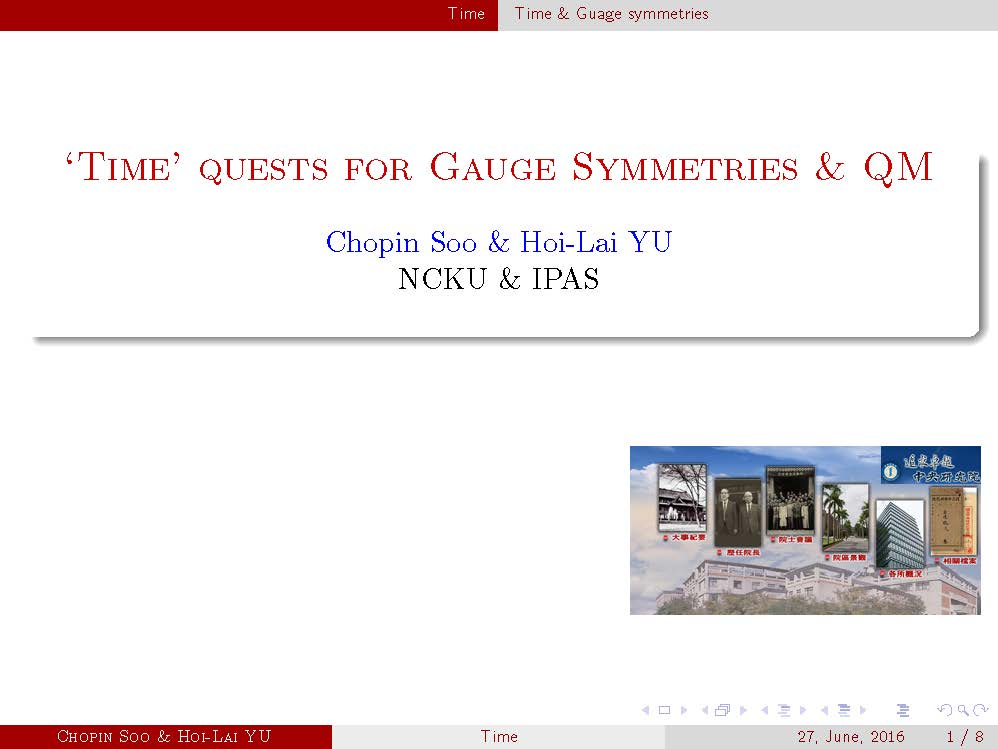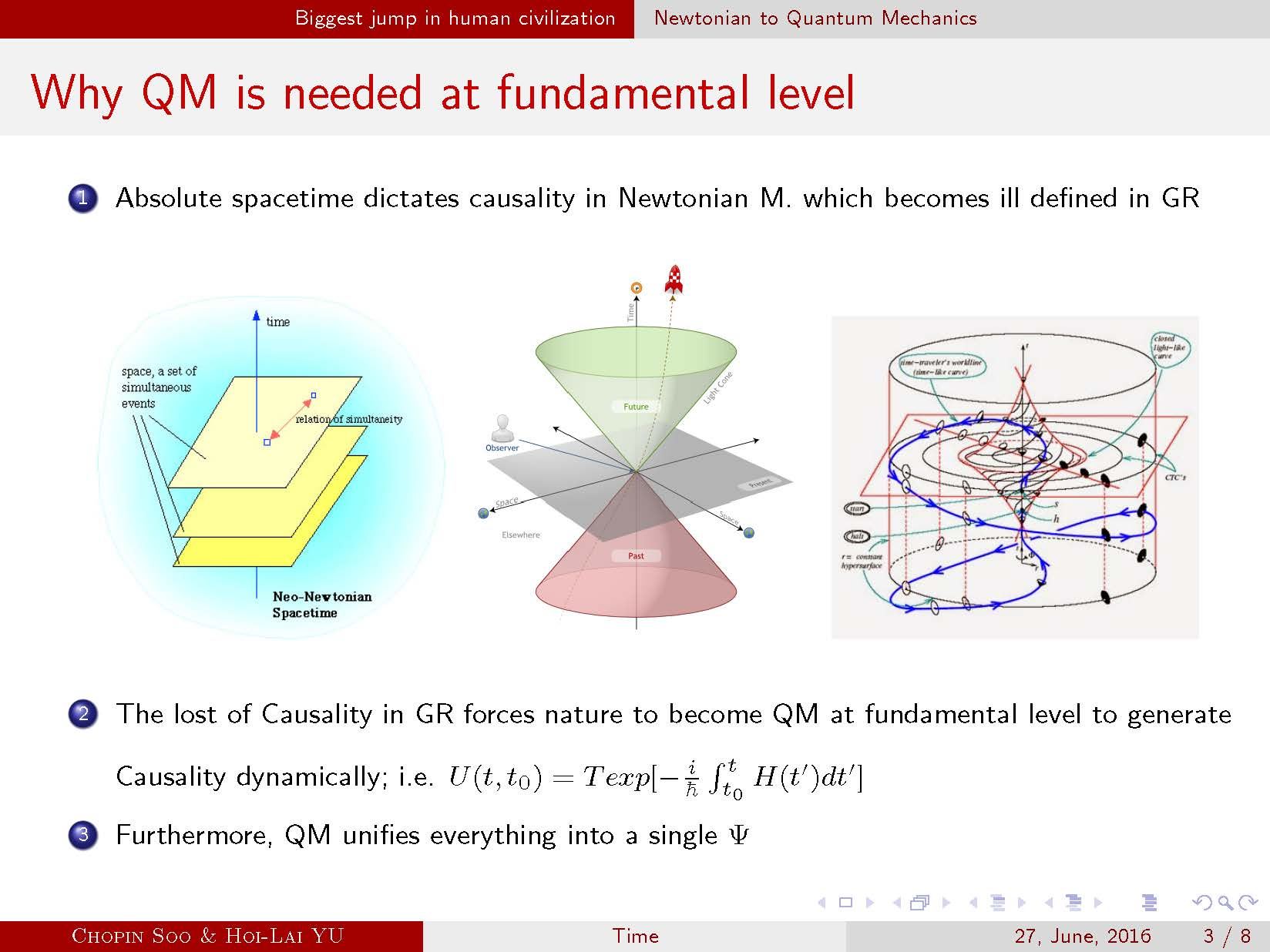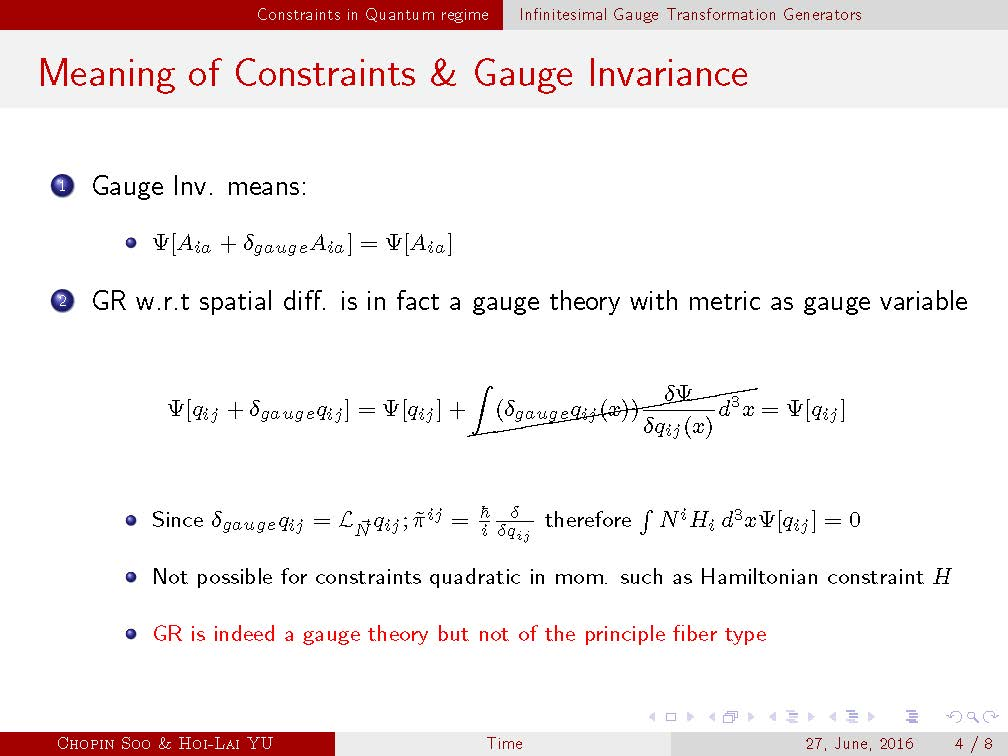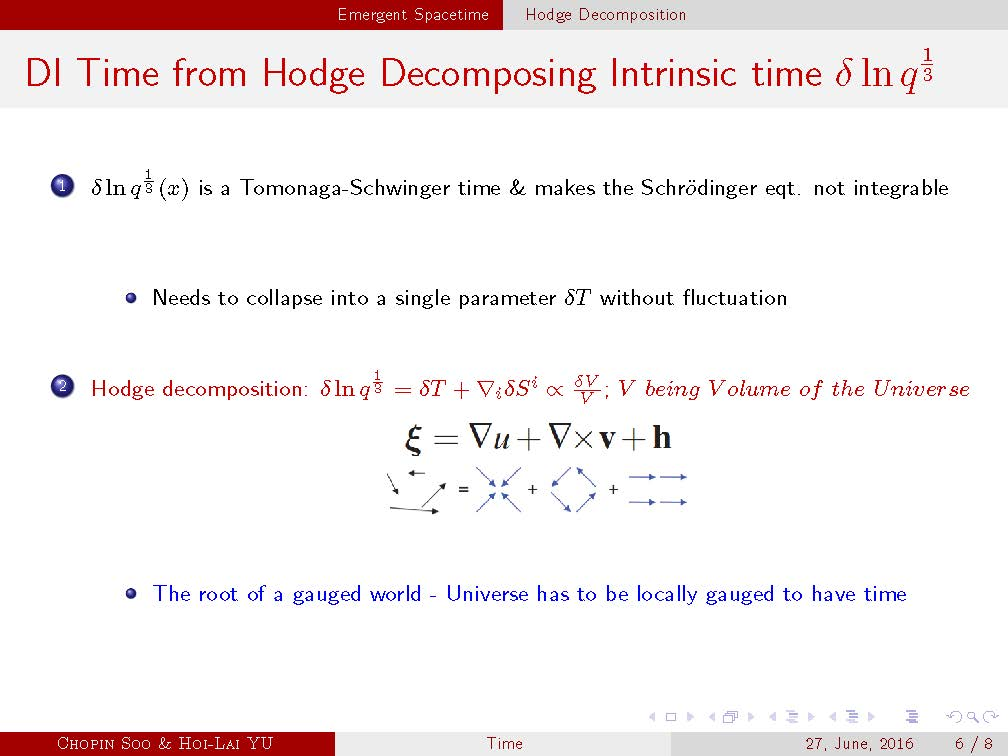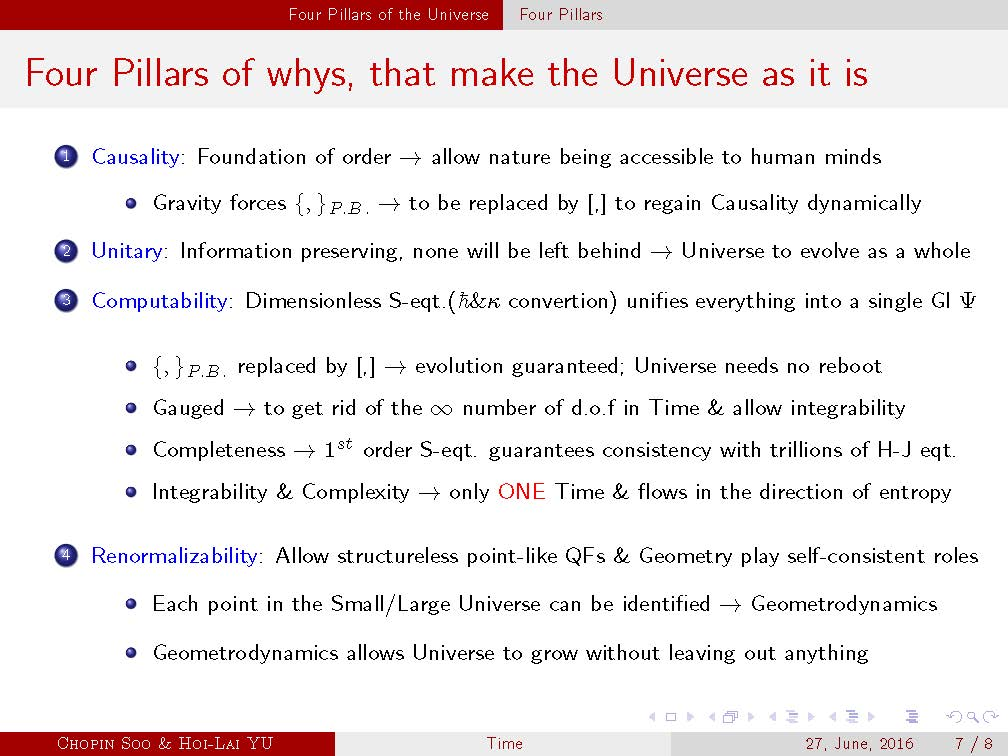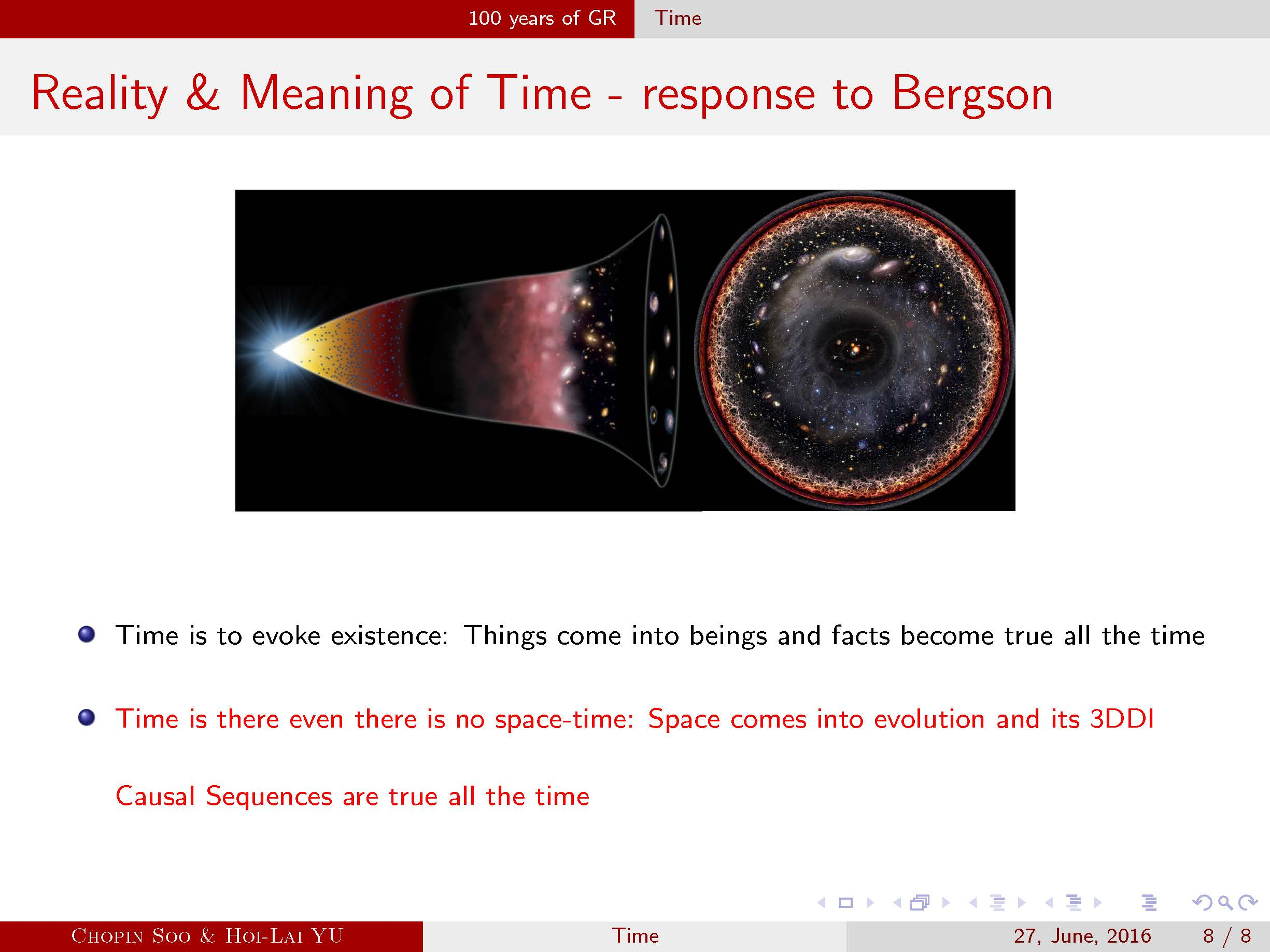Here are the links to some of our research works:

Intrinsic Time Gravity and the Lichnerowicz-York Equation, Niall OMurchadha, Chopin Soo and Hoi-Lai Yu; Class. Quantum Grav. 30 (2013) 095016(Editorial Board Highlight of the year)

General Relativity without paradigm of space-time covariance, and resolution of the problem of time, Chopin Soo and Hoi Lai Yu; Prog. Theor. Exp. Phys. 2014, 013E01

Intrinsic time quantum geometrodynamics, Eyo Ita, Chopin Soo and Hoi Lai Yu; Prog. Theor. Exp. Phys. 2015, 083E01

New Commutation Relations for Quantum Gravity, Chopin Soo and Hoi Lai Yu; Chinese J. Phys., 53(2015), 110106-1(Special issues on the occasion of the hundredth years of GR)

New Looks at Gauge Theories and Quantum Gravity, Hoi Lai Yu(Invited talk at LeCospa ll, 2015)